# LOW-DENSITY PARITY-CHECK CODED SIGNALING OVER A PARALLEL MULTICHANNEL 4

NOTE: This section is Under-Edit if necessary: Construction began on September 27, 2022 and was finished on September 29, 2022.

Low-Density Parity-Check (LDPC) Binary Codes: Signaling over a Parallel MultiChannel with Fading and Iterative Message-Passing Channel Decoding

```by Darrell A. Nolta
September 29, 2022
```

The AdvDCSMT1DCSS (T1) Professional (T1 Version 2) system tool has been used to investigate the Bit Error Rate (BER) performance for Regular Gallager Coded Signaling over a Discrete-Time (DT) Parallel MultiChannel (PMC) with Rayleigh Fading and Additive White Gaussian Noise (AWGN). As a reminder, note that Regular Gallager Codes are Low-Density Parity-Check (LDPC) Codes. Multiple Iteration Soft Input/Soft-Decision Output (SISO) LDPC Code Channel Decoding using the Sum-Product Algorithm (SPA) is used to decode the output of the simulated DT PMC.

T1 Version 2 has been revised to supports decoding of LDPC Coded M-ary Signaling over an Additive White Gaussian Noise Memoryless PMC with or without Rayleigh or Rician Fading and with AWGN; and Discrete MultiTone (DMT) Modulation MultiCarrier/MultiChannel [Orthogonal frequency-division Multiplexing (OFDM) FFT-Based] with or without Rayleigh or Rician Fading and with AWGN.

Note that the subject of LDPC Coded Signaling over a Parallel MultiChannel with Fading is quite complicated and requires careful study of LDPC Codes, Parallel MultiChannels [especially the FFT-Based DMT Modulation (OFDM) PMC], and Fading (Rayleigh & Rician). A number of references was used to implement the topic of this paper in T1V2: they include Dr. Gallager's work on LDPC Codes (1962 paper  and 1963 book ); wireless communications and OFDM (1985 paper  & 2012 paper ); and OFDM Systems and Rayleigh Fading (2005 paper  & 2017 paper ).

This investigation/experiment was easily conducted by AdvDCSMT1DCSS (T1) Professional (T1 V2) because of the capabilities of T1V2 that are discussed in the paper 'Low-Density Parity-Check (LDPC) Binary Codes: Signaling over a Parallel MultiChannel and Iterative Message-Passing Channel Decoding.' This paper is found on this website.

As a reminder, a Low-Density Parity-Check Coded Parallel MultiChannel (MC) is partitioned into G parallel subchannel groups where a subchannel group consists of K parallel subchannels. The set {G * Ng} represents the possible partitions of the LDPC Code's blocklengh (N). This approach is used for the LDPC Code & Signaling over a PMC application because N can be very large and the process of Codebits to Channel Input Bits assignment can quickly become unmanageable. Note {li} is the Group's set of the Number of Channel Input Bits.

A Low-Density Parity-Check (LDPC) Coded AWGN Memoryless Parallel MultiChannel or DMT Modulation Parallel MultiCarrier Channel can be selected in T1V2 to experience Rayleigh or Rician Fading impairment. These multichannels support Coherent Signaling only (prior to a Fade event) and possess UnRestricted Bandwidth. This fading can exist in the parallel multichannels in one of two possible forms: 1) Fading exists in the SET of ALL SUBCHANNELS [AWGN Memoryless (ML) PMC] or SUBCARRIERS (DMT Modulation PMC); or 2) Fading exists in a SUBSET of SUBCHANNELS (AWGN Memoryless PMC) or SUBCARRIERS (DMT Modulation PMC). And the demodulation of the subchannel or subcarrier output of these parallel multichannels with fading can be performed without or with perfect Channel Side Information (CSI).

For Rayleigh or Rician Fading, the first form [Fading exists in the SET of ALL SUBCHANNELS (AWGN ML PMC) or SUBCARRIERS (DMT Modulation PMC)], the Fading normalized 'Energy Gain' Distribution (Distrb) LDPC Coded PMC) Groups Type can be one of two possible subtypes:

1) Rayleigh/Rician Fading 'Energy Gain': Constant Distribution over all of the PMC Groups, or

2) Rayleigh/Rician Fading 'Energy Gain': Gaussian Distribution over all of the PMC Groups.

For each PMC Group, the Fading 'Energy Gain' Distrb LDPC Coded Parallel Multiple Channel SubChannel Type can be one of two possible subtypes:

1) Constant Distribution over all of the SubChannels of a PMC Group; or

2) Gaussian Distribution over all of the SubChannels of a PMC Group.

Note that a PMC Group is identified by its Group Index Number (No.) and a PMC SubChannel of a Group is identified by its Group and SubChannel Index Numbers. The PMC Group or SubChannel indices are Zero-based numbers.

The User-specified Rayleigh or Rician Fading normalized 'Energy Gain' (dB) is specified at the Group level as the Baseband Fading Average (Avg) Received SNR to Transmitted SNR (Es/N0) Ratio dB as a constant value or Gaussian Group Mean value.

Note for Rician Fading, the Fading Component's Mean (gamma) as a Fraction of its Standard Deviation is also specified by the User.

For the Gaussian Distribution over all of the PMC Groups, the Group Gaussian Mean can be chosen to be one of three possible Group No. Mean types depending on the even/odd characteristic of the Number of PMC Groups:

1) Smallest Group No. (0); 2) Largest Group No.; or 3) Middle Group No..

The Group Mean Number type 1 or 2 is possible for the Even Number case and type 1, 2, or 3 is possible for the Odd Number case.

The User will specify the Fading PMC Groups 'Energy Gain' Gaussian Distribution Group Standard Deviation that is dependent on the number of Groups.

For the Gaussian Distribution over all of the PMC Group SubChannels, the Group SubChannel Gaussian Mean can be chosen to be one of three possible types depending on the even/odd characteristic of the Number of Subchannels of a PMC Group:

1) Smallest SubChannel No. (0); 2) Largest SubChannel No.; or 3) Middle SubChannel No..

The Group SubChannel Mean Number type 1 or 2 is possible for the Even Number case and type 1, 2, or 3 is possible for the Odd Number case.

Note all of the PMC Groups contain the same number of SubChannels.

The User will specify the Fading PMC Group Subchannel 'Energy Gain' Gaussian Distribution Group Subchannel Standard Deviation that is dependent on the number of Group SubChannels.

For Rayleigh or Rician Fading, the second form [Fading exists in a SUBSET of SUBCHANNELS (AWGN ML PMC) or SUBCARRIERS (DMT Modulation PMC)] allows the User to specify a randomly determined subset of SubChannels of a Group for all Groups that will experience Fading. The indices of these Fading Group SubChannels are selected via a Randomly Uniform Generation process. The User will specify the Number of Group SubChannels that are subjected to the Fading.

Note the Number of Fading SubChannels can be selected to be one to the total number of SubChannels for a Group.

The User will specify the Random Number Generation Seed (a Positive No.). Next, the User will decide the Number of Random Numbers to be generated for the Fading SubChannel Distribution prior to its generation. This number choice can be Default No. (100 Thousand) or User Specified. Then, the User will decide on the Maximum Number of Random Numbers that are necessary for the Number of Fading Group SubChannels Indices to be generated as specified by the User. This number can be Default No. (100 Thousand) or User Specified.

After the Distribution of Fading Group SubChannels has been selected, the User will then specify the normalized Baseband 'Energy Gain' [Avg Received SNR to Transmitted SNR (Es/N0) Ratio, in dB due to Fading which will be the same (constant) for all of the randomly selected Rayleigh or Rician Fading SubChannels for a Group.

Note for Rician Fading, the Fading Component's Mean (gamma) as a Fraction of its Standard Deviation is also specified by the User.

In the cases of LDPC Coded FFT-based DMT Modulation Parallel MultiChannel/MultiCarrier (MC) Channel with Fading, Cyclic Prefix (CP) Append option can be chosen. The CP type is DMT Symbol-based. And its Cyclic Prefix Length is User-Specified with its maximum length equal to the number of FFT samples for the particular FFT-based DMT Modulation MC Channel. Further, the FFT-based DMT Modulation MC Channel with Fading is simulated using the Linear Convolution or Cyclic (Circular) Convolution method (User-Specified).

The purpose of this investigation/experiment is to obtain via T1 V2 the simulated BER results of (N = 504, j = 3, k = 4) Regular Gallager Coded Signaling over a selected PMC that experiences Rayleigh Fading in certain subchannels and SPA Decoding using Channel Side Information Demodulation. For this particular Regular Gallager Code (RGC), its corresponding DT Waveform (DTW) AWGN ML PMC or DT DMT Modulation PMC has 96 subchannels or 96 subcarriers/subchannels, respectively. For this experiment, we consider the cases where Rayleigh Fading exists in all subchannels (96) or in one (1), three (3), six (6), 12, 24, or 48 subchannels. This is the set of number of Rayleigh Fading subchannels: {No. SubC: 1, 3, 6, 12, 24, 48, 96}. Next using the selected PMC Fading simulated BER results we compare graphically the Rayleigh Fading No. SubC subsets results for each simulated PMC. The reference for these results is (N = 504, j = 3, k = 4) Regular Gallager Coded Signaling over a DTW AWGN ML or DT DMT Modulation PMC without Rayleigh Fading and SPA decoding. Then we compare the DMT Modulation PMC simulated BER results versus AWGN ML PMC simulated BER results for each Rayleigh Fading No. SubC subset.

Note that for this experiment, T1 V2 used the DMT Modulation PMC MultiCarrier Signal transmitted over a Single Channel model (DMT Modulation PMC Type 0). And the Cyclic Prefix (CP) is used with a CP Length of 64 (a quarter of 256 Inverse FFT Samples). Further, the T1 V2 simulated BER results are based on that the SPA Decoder having access to perfect Channel Side Information, i.e., the Rayleigh Fading parameters are known by the SPA Decoder.

Consider the SPA Decoding Algorithm Bit Error Rate (BER) or Bit Error Probability Pb performance simulation results for Regular Gallager Coded (RGC) Generator-based Encoding and Signaling that were produced by T1 V2 that are displayed below in Figure 1 plot for the AWGN ML PMC with Rayleigh Fading for one (1), 3, 6, 12, 24, 48, and 96 subchannels; Figure 2 plot for DMT Modulation PMC with Rayleigh Rading for one (1), three (3), six (6), 12, 24, 48, and 96 subchannels/subcarriers, respectively. Further consider Figure 3, 4, 5, 6, 7, 8, and 9 plots that show the simulated BER results for the AWGN ML PMC and DMT Modulation PMC with 96, 48, 24, 12, 6, 3, 1 Rayleigh Fading subchannels, respectively.

Each figure's BER plot displays eight or four curves where a curve is constructed from the set of simulated Pb values that correspond to a set of Eb/N0 [Signal-to-Noise Ratio (SNR)] values. Thus, a BER curve is represented as {(Eb/N0, Pb)} for a number of T1 V2 simulations. Each RGC curve displays the BER performance behavior of a Regular Gallager Coding and SPA Iterative Decoding system example that was used for a T1 V2 simulation.

Since a PMC simulation consists of a number of Signaling Schemes (Distinct) the possible choices for the set of Signal Scheme's Eb/N0(k) values can become very large. To simplify this matter, for each Pb simulation, all the Signaling Schemes' Eb/N0(k) values are specified so that they are all equal. Thus, a plot's Eb/N0 value is defined as

Eb/N0 = Eb/N0(1) = Eb/N0(2) = … = Eb/N0(K) , for 1 through K Signaling Schemes.

The Gallager LDPC Coding System that were simulated to produce BER results for the DTW AWGN ML PMC or DT DMT Modulation PMC (FFT-Based) (as shown in Figure 1 - 9) are as follows:

[Number of Code Word Bits (N) = 504, column weight (j) = 3, row weight (k) = 4] Regular Gallager Code [Rate (R) = 0.253968], {G * Ng} = {12 * 42}, 96 Subchannels, Distinct 8-MC Group (G), {li} = {1,1,2,6,8,8,8,8} <=> {BPSK, PI/2 BPSK, QPSK, 64-QAM, 256-QAM, 256-QAM, 256-QAM, 256-QAM} for 1,000,064 Equal probable Independent and Identical Distributed (I.I.D.) Information (Info) Bits.

Each DTW AWGN ML PMC subchannel uses a Half-Cosine Modulator and Demodulator with eight (8) samples per waveform.

The DT FFT-Based DMT Modulation PMC is based on 256 IFFT Samples per Frame (Block).

The DTW AWGN ML PMC or DT DMT Modulation PMC has Non Distorting, UnRestricted Bandwidth.

The Frequency Non-Selective Rayleigh Fading in each fading subchannel of DTW AWGN ML PMC is based on a -5.25 dB Normalized 'Energy Gain.'

The Frequency Selective Rayleigh Fading in each fading subcharrier/subchannel of DT FFT-Based DMT Modulation PMC is based on a -5.25 dB Normalized 'Energy Gain.'

Sum-Product Algorithm (SPA) Iterative Decoder is using Model 2 (Check Messages then Bit Messages Iteration Processing), Theoretical SPA Implementation Type, and Maximum Number of Iterations per Block (Imax) = 50.

Figure 1 displays the BER versus Eb/N0 for the Maximum Number of Iterations per Block (Imax) of 50 for the above described Gallager LDPC Coding System for Signaling over a DTW AWGN ML PMC (Non Distorting, UnRestricted Bandwidth) with Rayleigh Fading in 96 (all), 48, 24, 12, 6, 3, 1 subchannel (s) and using SPA Decoding with perfect CSI demodulation.

Figure 2 displays the BER versus Eb/N0 for the Maximum Number of Iterations per Block (Imax) of 50 for the above described Gallager LDPC Coding System for Signaling over LDPC Coded FFT-based DMT Discrete Time Waveform AWGN Modulation Channel Type 0 (MultiCarrier Signal transmitted over a Single Channel) with Rayleigh Fading in 96 (all), 48, 24, 12, 6, 3, 1 subchannel (s) and using SPA Decoding with perfect CSI demodulation.

Figure 3 displays the BER versus Eb/N0 for the Maximum Number of Iterations per Block (Imax) of 50 for the above described Gallager LDPC Coding System for Signaling over a DTW AWGN ML PMC or DT DMT Modulation PMC with Rayleigh Fading in all subchannels (96) and using SPA Decoding with perfect CSI demodulation.

Figure 4, 5, 6, 7, 8, and 9 displays the BER versus Eb/N0 for the Maximum Number of Iterations per Block (Imax) of 50 for the above described Gallager LDPC Coding System for Signaling over a DTW AWGN ML PMC or DT DMT Modulation PMC with Rayleigh Fading in 48, 24, 12, 6, 3 ,1 subchannels, respectively, and using SPA Decoding with perfect CSI demodulation.

There are a number of important conclusions that can be drawn from the below displayed simulated Iterative LDPC Code SPA Decoding BER results in Figure 1, 2, 3, 4, 5, 6, 7, 8, and 9. It appears that T1 V2 is correctly modeling and simulating the SPA Decoding (with perfect Channel Side Information Demodulation) of LDPC Coded Signaling over a Parallel MultiChannel/MultiCarrier Channel with Rayleigh Fading and with AWGN for the selected Regular Gallager Code.

In regards to the Figures 1 through 9 simulated BER curve references, we have:

1) (N = 504, j = 3, k = 4) Regular Gallager Coded Signaling over DTW AWGN ML PMC without Rayleigh Fading and SPA Decoding, we see in Figure 1 through 9 the expected behavior (the steep reduction in BER as the SNR is increased between 7 dB and 9 dB).

2) (N = 504, j = 3, k = 4) Regular Gallager Coded Signaling over DT DMT Modulation PMC (FFT-Based) without Rayleigh Fading and SPA Decoding, we see in Figure 2 through 9 the expected behavior (the steep reduction in BER as the SNR is increased between 7 dB and 9 dB).

Note that these two simulated BER curves are almost identical.

Figure 1 clearly shows the bad simulated BER performance when Rayleigh Fading exists in all of the subchannels of a DTW AWGN ML PMC. But this simulated BER performance does begin to slightly improve at the high end of the Eb/N0 range (14 dB). As expected, as one decreases the number of subchannels that experience Rayleigh Fading, the BER performance improves and approaches the BER performance of this RGC PMC with no Rayleigh Fading. But even when only one subchannel experiences Rayleigh Fading, the simulated BER performance does not match the case where no Rayleigh Fading exists in the PMC.

Figure 2 clearly shows the bad simulated BER performance when Rayleigh Fading exists in all of the subchannels of a DT FFT-Based DMT Modulation PMC. This simulated BER performance stays flat throughout the Eb/N0 range (2 - 14 dB). As one decreases the number of subchannels that experience Rayleigh Fading, the BER performance improves at the high end of Eb/N0 range but does not approaches the BER performance of this RGC PMC with no Rayleigh Fading. But only one case (1 Rayleigh Fading subchannel) simulated BER performance goes to zero (0) at Eb/N0 of 14 dB.

Figure 3 clearly shows that when all of the subchannels experience Rayleigh Fading for DTW AWGN ML or DT DMT Modulation PMC, the simulated BER performance is very bad. The DT DMT Modulation PMC BER curve is flat (greater than 0.1 while the DTW AWGN ML PMC BER curve begin to bend down under 10-1 for Eb/N0 of 14 dB.

Figure 4 clearly shows that when 48 subchannels experience Rayleigh Fading for DT DMT Modulation PMC the simulated BER performance is very bad (essential flat curve & > 10-1). But when 48 subchannels of DTW AWGN ML PMC experience Rayleigh Fading, the simulated BER performance does go to zero at the high end of the Eb/N0 range. But the BER curve for DTW AWGN ML PMC with Rayleigh Fading does not come close to matching BER curve for the corresponding case without Rayleigh Fading.

Figure 5 clearly shows that when 24 subchannels experience Rayleigh Fading for DT DMT Modulation PMC the simulated BER performance is very bad (essential flat curve & > 10-1). But when 24 subchannels of DTW AWGN ML PMC experience Rayleigh Fading, the simulated BER performance does go to zero at the middle portion of the Eb/N0 range. But the BER curve for DTW AWGN ML PMC with Rayleigh Fading does starts to match the BER curve for the corresponding case without Rayleigh Fading.

Figure 6 clearly shows that when 12 subchannels experience Rayleigh Fading for DT DMT Modulation PMC the simulated BER performance is bad (curve descends & flattens out with Pb > 10-2). But when 12 subchannels of DTW AWGN ML PMC experience Rayleigh Fading, the simulated BER performance does go to zero at the middle portion of the Eb/N0 range. The BER curve for DTW AWGN ML PMC with Rayleigh Fading does approach the BER curve for the corresponding case without Rayleigh Fading.

Figure 7 clearly shows that when 6 subchannels experience Rayleigh Fading for DT DMT Modulation PMC the simulated BER performance is getting better (curve descends & flattens out with Pb > 10-3). But when 6 subchannels of DTW AWGN ML PMC experience Rayleigh Fading, the simulated BER performance does go to zero at the middle portion of the Eb/N0 range. The BER curve for DTW AWGN ML PMC with Rayleigh Fading does approach the BER curve for the corresponding case without Rayleigh Fading.

Figure 8 clearly shows that when 3 subchannels experience Rayleigh Fading for DT DMT Modulation PMC the simulated BER performance is getting better (curve descends to Pb > 10-4). But when 3 subchannels of DTW AWGN ML PMC experience Rayleigh Fading, the simulated BER performance does go to zero at the middle portion of the Eb/N0 range. The BER curve for DTW AWGN ML PMC with Rayleigh Fading is closer to the BER curve for the corresponding case without Rayleigh Fading.

Figure 9 clearly shows that when one (1) subchannel experiences Rayleigh Fading for DT DMT Modulation PMC the simulated BER performance is getting better (curve descends to Pb = 0 at Eb/N0 of 14 dB). But when 1 subchannel of DTW AWGN ML PMC experiences Rayleigh Fading, the simulated BER performance does go to zero at the middle portion of the Eb/N0 range. The BER curve for DTW AWGN ML PMC with Rayleigh Fading is very close to the BER curve for the corresponding case without Rayleigh Fading.

Based on the T1 V2 simulated BER results comparisons as shown from Figure 3 through 9, the DTW AWGN ML PMC is better to signal over as compared to the DT FFT-Based DMT Modulation PMC when each PMC experiences Rayleigh Fading for the selected (N = 504, j = 3, k = 4) Regular Gallager Coding System and SPA Decoding with perfect CSI demodulation.

T1 Professional (T1 V2) now offers the LDPC Code (Gallager, Array, and Repeat-Accumulate) construction and LDPC Channel Coding and LDPC Channel Decoding (Iterative; based on the 'Symbol-by-Symbol' MAP Belief Propagation algorithm) for Parallel MultiChannel (PMC) features to the User. The User can choose the simulated Signaling Channel as Additive White Gaussian Noise (AWGN) PMC with AWGN, Crosstalk (XTALK) PMC, or Discrete MultiTone (DMT) Modulation PMC with AWGN. Further, the user can choose the simulated PMC to possess a NonDistorting, UnRestricted Bandwidth or a Distorting, Restricted Bandwidth. And, the User can choose to add Rayleigh or Rician Fading to the AWGN PMC or DMT Modulation PMC with NonDistorting, UnRestricted Bandwidth.

An important note to be recognized is that the DMT Modulation PMC is also known as Orthogonal Frequency-Division Multiplexing (OFDM). T1 V2's OFDM implementation is FFT-Based.

Also, it is important to realize that New Radio (NR), a Fifth-Generation (5G) Telecommunications Technologies, has been proposed to use LDPC Channel Codes  & Cyclic Prefix (CP) OFDM (FFT-based)-based waveforms.

T1 Professional will provide the User the introductory opportunity to study the complexity of BER performance of the Low-Density Parity-Check Coded M-ary Signal over an OFDM (FFT-based) MultiCarrier/MultiChannel (with CP for Distorting, Restricted Bandwidth PMC or No CP for a NonDistorting, UnRestricted Bandwidth PMC) and Soft-Decision Decoding using the Sum-Product Algorithm.

In conclusion, the User via T1 V2 can get experience with the Generation of LDPC codes and the Sum-Product and Bit Flipping algorithms as applied to Iterative Decoding in simulated digital communication systems for Spacecraft and Mobile Communications and Digital Storage Systems LDPC Coding applications.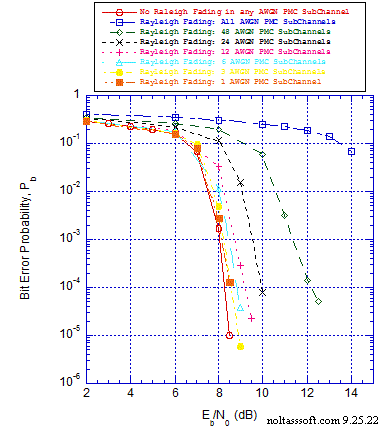```Figure 1. Bit Error Probability for (N = 504, j = 3, k = 4) Gallager Coded Signaling
over a Discrete-Time Waveform (DTW) AWGN Parallel MultiChannel (PMC) without or
with Rayleigh Fading & with AWGN:
```
```Equal Probable Independent and Identical Distributed (I.I.D.) Source for 1,000,064
Information Bits;
```
```N = 504, j = 3, k = 4, L = 128, Rate = 0.253968 Regular Gallager Code (T1V2
Computer-Generated;
```
```The Distinct 8-MC Group Signaling Schemes consist of {li} = {1,1,2,6,8,8,8,8} <=>
{BPSK, PI/2 BPSK,QPSK,64-QAM,256-QAM,…,256-QAM};
```
```For each simulated Pb value, Eb/N0 = Eb/N0(1) = Eb/N0(2) = … = Eb/N0(K), for 1 through
K Signaling Schemes;
```
```96 DTW subchannels: Each Gallager Coded DTW AWGN PMC subchannel consists of
half-cosine orthonormal baseband shaping pulse, 8 symbols per symbol period, and
half-cosine matched filter demodulator front-end;
```
```These DTW subchannels possess a NonDistorting, UnRestricted Bandwidth;
```
```Rayleigh Fading: -5.25 dB Normalized Energy Gain for a fading subchannel; &
```
```Sum-Product Algorithm Iterative Decoder using Model 2 (Check Messages then Bit
Messages Iteration Processing), Theoretical SPA Implementation Type, and Maximum
Number of Iterations per Block (Imax) = 50; and Perfect Channel Side Information
Demodulation for AWGN PMC with Rayleigh Fading.
```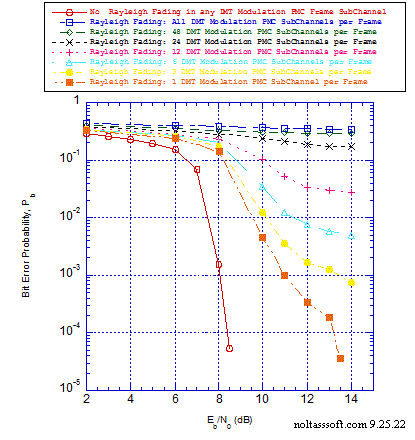```Figure 2. Bit Error Probability for (N = 504, j = 3, k = 4, Gallager Coded Signaling
over a Discrete-Time (DT) FFT-Based DMT Modulation Parallel MultiChannel (PMC)
without or with Rayleigh Fading & with AWGN:
```
```Equal Probable Independent and Identical Distributed (I.I.D.) Source for 1,000,064
Information Bits;
```
```N = 504, j = 3, k = 4, L = 128, Rate = 0.253968 Regular Gallager Code (T1V2
Computer-Generated;
```
```The Distinct 8-MC Group Signaling Schemes consist of {li} = {1,1,2,6,8,8,8,8} <=>
{BPSK, PI/2 BPSK,QPSK,64-QAM,256-QAM,…,256-QAM};
```
```For each simulated Pb value, Eb/N0 = Eb/N0(1) = Eb/N0(2) = … = Eb/N0(K), for 1 through
K Signaling Schemes;
```
```96 DT FFT-Based DMT Modulation SubCarriers/SubChannels & 256 IFFT Samples per Frame;
```
```These DTW subchannels possess a NonDistorting, UnRestricted Bandwidth;
```
```Frequency-Selective Rayleigh Fading: -5.25 dB Normalized Energy Gain for a subcarrier
```
```Cyclic Prefix (CP) added for Rayleigh Fading cases (CP Length = 64 samples); &
```
```Sum-Product Algorithm Iterative Decoder using Model 2 (Check Messages then Bit
Messages Iteration Processing), Theoretical SPA Implementation Type, and Maximum
Number of Iterations per Block (Imax) = 50; and Perfect Channel Side Information
Demodulation for DMT Modulation PMC with Rayleigh Fading.
```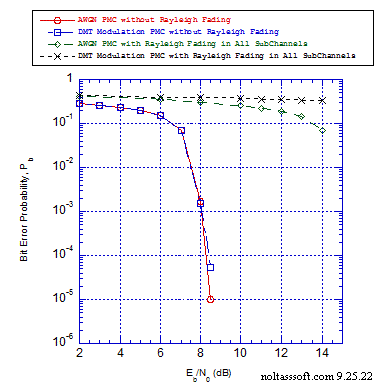```Figure 3. Bit Error Probability for (N = 504, j = 3, k = 4, Gallager Coded Signaling
over a Discrete-Time Waveform (DTW) AWGN or FFT-Based DMT Modulation (Mod) Parallel
MultiChannel (PMC) without or with Rayleigh Fading (All SubChannels) & with AWGN:
```
```Equal Probable Independent and Identical Distributed (I.I.D.) Source for 1,000,064
Information Bits;
```
```N = 504, j = 3, k = 4, L = 128, Rate = 0.253968 Regular Gallager Code (T1V2
Computer-Generated;
```
```The Distinct 8-MC Group Signaling Schemes consist of {li} = {1,1,2,6,8,8,8,8} <=>
{BPSK, PI/2 BPSK,QPSK,64-QAM,256-QAM,…,256-QAM};
```
```For each simulated Pb value, Eb/N0 = Eb/N0(1) = Eb/N0(2) = … = Eb/N0(K), for 1 through
K Signaling Schemes;
```
```96 DTW AWGN PMC subchannels: Each Gallager Coded subchannel consists of half-cosine
orthonormal baseband shaping pulse, 8 symbols per symbol period, and half-cosine
matched filter demodulator front-end;
```
```96 DT FFT-Based DMT Mod PMC SubCarriers/SubChannels & 256 IFFT Samples per Frame;
```
```These AWGN or DMT Mod PMC subchannels possess a NonDistorting, UnRestricted Bandwidth;
```
```Rayleigh Fading for AWGN PMC: -5.25 dB Normalized Energy Gain for a fading subchannel;
```
```Frequency-Selective Rayleigh Fading for DMT Mod PMC: -5.25 dB Normalized Energy Gain
```
```Cyclic Prefix (CP) added for Rayleigh Fading DMT Mod PMC cases (CP Length = 64 samples); &
```
```Sum-Product Algorithm Iterative Decoder using Model 2 (Check Messages then Bit
Messages Iteration Processing), Theoretical SPA Implementation Type, and Maximum
Number of Iterations per Block (Imax) = 50; and Perfect Channel Side Information
Demodulation for PMC with Rayleigh Fading.
```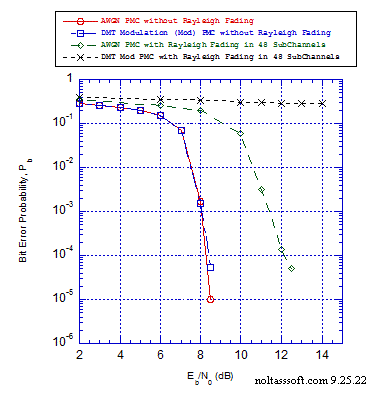```Figure 4. Bit Error Probability for (N = 504, j = 3, k = 4, Gallager Coded Signaling
over a Discrete-Time Waveform (DTW) AWGN or FFT-Based DMT Modulation (Mod) Parallel
MultiChannel (PMC) without or with Rayleigh Fading (48 SubChannels) & with AWGN:
```
```Equal Probable Independent and Identical Distributed (I.I.D.) Source for 1,000,064
Information Bits;
```
```N = 504, j = 3, k = 4, L = 128, Rate = 0.253968 Regular Gallager Code (T1V2
Computer-Generated;
```
```The Distinct 8-MC Group Signaling Schemes consist of {li} = {1,1,2,6,8,8,8,8} <=>
{BPSK, PI/2 BPSK,QPSK,64-QAM,256-QAM,…,256-QAM};
```
```For each simulated Pb value, Eb/N0 = Eb/N0(1) = Eb/N0(2) = … = Eb/N0(K), for 1 through
K Signaling Schemes;
```
```96 DTW AWGN PMC subchannels: Each Gallager Coded subchannel consists of half-cosine
orthonormal baseband shaping pulse, 8 symbols per symbol period, and half-cosine
matched filter demodulator front-end;
```
```96 DT FFT-Based DMT Mod PMC SubCarriers/SubChannels & 256 IFFT Samples per Frame;
```
```These AWGN or DMT Mod PMC subchannels possess a NonDistorting, UnRestricted Bandwidth;
```
```Rayleigh Fading for AWGN PMC: -5.25 dB Normalized Energy Gain for a fading subchannel;
```
```Frequency-Selective Rayleigh Fading for DMT Mod PMC: -5.25 dB Normalized Energy Gain
```
```Cyclic Prefix (CP) added for Rayleigh Fading DMT Mod PMC cases (CP Length = 64 samples); &
```
```Sum-Product Algorithm Iterative Decoder using Model 2 (Check Messages then Bit
Messages Iteration Processing), Theoretical SPA Implementation Type, and Maximum
Number of Iterations per Block (Imax) = 50; and Perfect Channel Side Information
Demodulation for PMC with Rayleigh Fading.
```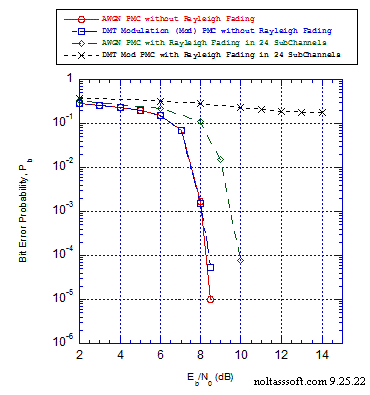```Figure 5. Bit Error Probability for (N = 504, j = 3, k = 4, Gallager Coded Signaling
over a Discrete-Time Waveform (DTW) AWGN or FFT-Based DMT Modulation (Mod) Parallel
MultiChannel (PMC) without or with Rayleigh Fading (24 SubChannels) & with AWGN:
```
```Equal Probable Independent and Identical Distributed (I.I.D.) Source for 1,000,064
Information Bits;
```
```N = 504, j = 3, k = 4, L = 128, Rate = 0.253968 Regular Gallager Code (T1V2
Computer-Generated;
```
```The Distinct 8-MC Group Signaling Schemes consist of {li} = {1,1,2,6,8,8,8,8} <=>
{BPSK, PI/2 BPSK,QPSK,64-QAM,256-QAM,…,256-QAM};
```
```For each simulated Pb value, Eb/N0 = Eb/N0(1) = Eb/N0(2) = … = Eb/N0(K), for 1 through
K Signaling Schemes;
```
```96 DTW AWGN PMC subchannels: Each Gallager Coded subchannel consists of half-cosine
orthonormal baseband shaping pulse, 8 symbols per symbol period, and half-cosine
matched filter demodulator front-end;
```
```96 DT FFT-Based DMT Mod PMC SubCarriers/SubChannels & 256 IFFT Samples per Frame;
```
```These AWGN or DMT Mod PMC subchannels possess a NonDistorting, UnRestricted Bandwidth;
```
```Rayleigh Fading for AWGN PMC: -5.25 dB Normalized Energy Gain for a fading subchannel;
```
```Frequency-Selective Rayleigh Fading for DMT Mod PMC: -5.25 dB Normalized Energy Gain
```
```Cyclic Prefix (CP) added for Rayleigh Fading DMT Mod PMC cases (CP Length = 64 samples); &
```
```Sum-Product Algorithm Iterative Decoder using Model 2 (Check Messages then Bit
Messages Iteration Processing), Theoretical SPA Implementation Type, and Maximum
Number of Iterations per Block (Imax) = 50; and Perfect Channel Side Information
Demodulation for PMC with Rayleigh Fading.
```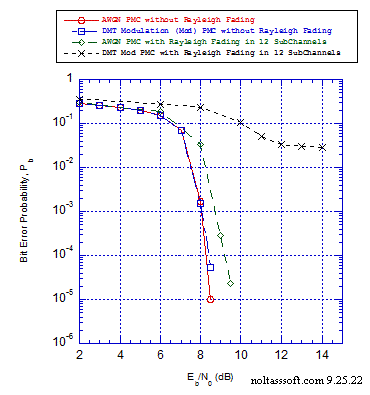```Figure 6. Bit Error Probability for (N = 504, j = 3, k = 4, Gallager Coded Signaling
over a Discrete-Time Waveform (DTW) AWGN or FFT-Based DMT Modulation (Mod) Parallel
MultiChannel (PMC) without or with Rayleigh Fading (12 SubChannels) & with AWGN:
```
```Equal Probable Independent and Identical Distributed (I.I.D.) Source for 1,000,064
Information Bits;
```
```N = 504, j = 3, k = 4, L = 128, Rate = 0.253968 Regular Gallager Code (T1V2
Computer-Generated;
```
```The Distinct 8-MC Group Signaling Schemes consist of {li} = {1,1,2,6,8,8,8,8} <=>
{BPSK, PI/2 BPSK,QPSK,64-QAM,256-QAM,…,256-QAM};
```
```For each simulated Pb value, Eb/N0 = Eb/N0(1) = Eb/N0(2) = … = Eb/N0(K), for 1 through
K Signaling Schemes;
```
```96 DTW AWGN PMC subchannels: Each Gallager Coded subchannel consists of half-cosine
orthonormal baseband shaping pulse, 8 symbols per symbol period, and half-cosine
matched filter demodulator front-end;
```
```96 DT FFT-Based DMT Mod PMC SubCarriers/SubChannels & 256 IFFT Samples per Frame;
```
```These AWGN or DMT Mod PMC subchannels possess a NonDistorting, UnRestricted Bandwidth;
```
```Rayleigh Fading for AWGN PMC: -5.25 dB Normalized Energy Gain for a fading subchannel;
```
```Frequency-Selective Rayleigh Fading for DMT Mod PMC: -5.25 dB Normalized Energy Gain
```
```Cyclic Prefix (CP) added for Rayleigh Fading DMT Mod PMC cases (CP Length = 64 samples); &
```
```Sum-Product Algorithm Iterative Decoder using Model 2 (Check Messages then Bit
Messages Iteration Processing), Theoretical SPA Implementation Type, and Maximum
Number of Iterations per Block (Imax) = 50; and Perfect Channel Side Information
Demodulation for PMC with Rayleigh Fading.
```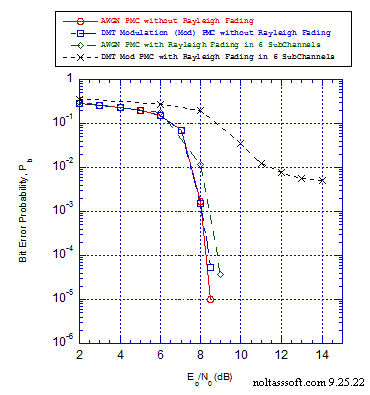```Figure 7. Bit Error Probability for (N = 504, j = 3, k = 4, Gallager Coded Signaling
over a Discrete-Time Waveform (DTW) AWGN or FFT-Based DMT Modulation (Mod) Parallel
MultiChannel (PMC) without or with Rayleigh Fading (6 SubChannels) & with AWGN:
```
```Equal Probable Independent and Identical Distributed (I.I.D.) Source for 1,000,064
Information Bits;
```
```N = 504, j = 3, k = 4, L = 128, Rate = 0.253968 Regular Gallager Code (T1V2
Computer-Generated;
```
```The Distinct 8-MC Group Signaling Schemes consist of {li} = {1,1,2,6,8,8,8,8} <=>
{BPSK, PI/2 BPSK,QPSK,64-QAM,256-QAM,…,256-QAM};
```
```For each simulated Pb value, Eb/N0 = Eb/N0(1) = Eb/N0(2) = … = Eb/N0(K), for 1 through
K Signaling Schemes;
```
```96 DTW AWGN PMC subchannels: Each Gallager Coded subchannel consists of half-cosine
orthonormal baseband shaping pulse, 8 symbols per symbol period, and half-cosine
matched filter demodulator front-end;
```
```96 DT FFT-Based DMT Mod PMC SubCarriers/SubChannels & 256 IFFT Samples per Frame;
```
```These AWGN or DMT Mod PMC subchannels possess a NonDistorting, UnRestricted Bandwidth;
```
```Rayleigh Fading for AWGN PMC: -5.25 dB Normalized Energy Gain for a fading subchannel;
```
```Frequency-Selective Rayleigh Fading for DMT Mod PMC: -5.25 dB Normalized Energy Gain
```
```Cyclic Prefix (CP) added for Rayleigh Fading DMT Mod PMC cases (CP Length = 64 samples); &
```
```Sum-Product Algorithm Iterative Decoder using Model 2 (Check Messages then Bit
Messages Iteration Processing), Theoretical SPA Implementation Type, and Maximum
Number of Iterations per Block (Imax) = 50; and Perfect Channel Side Information
Demodulation for PMC with Rayleigh Fading.
```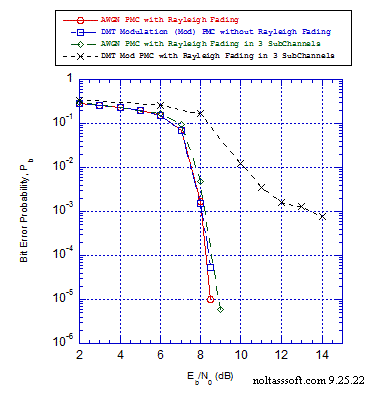```Figure 8. Bit Error Probability for (N = 504, j = 3, k = 4, Gallager Coded Signaling
over a Discrete-Time Waveform (DTW) AWGN or FFT-Based DMT Modulation (Mod) Parallel
MultiChannel (PMC) without or with Rayleigh Fading (3 SubChannels) & with AWGN:
```
```Equal Probable Independent and Identical Distributed (I.I.D.) Source for 1,000,064
Information Bits;
```
```N = 504, j = 3, k = 4, L = 128, Rate = 0.253968 Regular Gallager Code (T1V2
Computer-Generated;
```
```The Distinct 8-MC Group Signaling Schemes consist of {li} = {1,1,2,6,8,8,8,8} <=>
{BPSK, PI/2 BPSK,QPSK,64-QAM,256-QAM,…,256-QAM};
```
```For each simulated Pb value, Eb/N0 = Eb/N0(1) = Eb/N0(2) = … = Eb/N0(K), for 1 through
K Signaling Schemes;
```
```96 DTW AWGN PMC subchannels: Each Gallager Coded subchannel consists of half-cosine
orthonormal baseband shaping pulse, 8 symbols per symbol period, and half-cosine
matched filter demodulator front-end;
```
```96 DT FFT-Based DMT Mod PMC SubCarriers/SubChannels & 256 IFFT Samples per Frame;
```
```These AWGN or DMT Mod PMC subchannels possess a NonDistorting, UnRestricted Bandwidth;
```
```Rayleigh Fading for AWGN PMC: -5.25 dB Normalized Energy Gain for a fading subchannel;
```
```Frequency-Selective Rayleigh Fading for DMT Mod PMC: -5.25 dB Normalized Energy Gain
```
```Cyclic Prefix (CP) added for Rayleigh Fading DMT Mod PMC cases (CP Length = 64 samples); &
```
```Sum-Product Algorithm Iterative Decoder using Model 2 (Check Messages then Bit
Messages Iteration Processing), Theoretical SPA Implementation Type, and Maximum
Number of Iterations per Block (Imax) = 50; and Perfect Channel Side Information
Demodulation for PMC with Rayleigh Fading.
```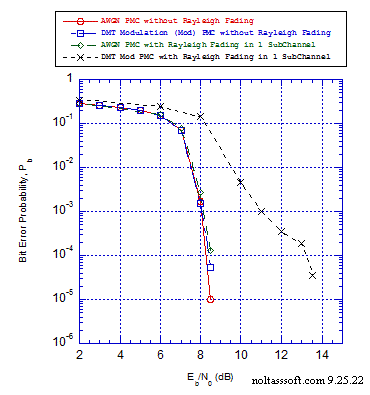```Figure 9. Bit Error Probability for (N = 504, j = 3, k = 4, Gallager Coded Signaling
over a Discrete-Time Waveform (DTW) AWGN or FFT-Based DMT Modulation (Mod) Parallel
MultiChannel (PMC) without or with Rayleigh Fading (1 SubChannel) & with AWGN:
```
```Equal Probable Independent and Identical Distributed (I.I.D.) Source for 1,000,064
Information Bits;
```
```N = 504, j = 3, k = 4, L = 128, Rate = 0.253968 Regular Gallager Code (T1V2
Computer-Generated;
```
```The Distinct 8-MC Group Signaling Schemes consist of {li} = {1,1,2,6,8,8,8,8} <=>
{BPSK, PI/2 BPSK,QPSK,64-QAM,256-QAM,…,256-QAM};
```
```For each simulated Pb value, Eb/N0 = Eb/N0(1) = Eb/N0(2) = … = Eb/N0(K), for 1 through
K Signaling Schemes;
```
```96 DTW AWGN PMC subchannels: Each Gallager Coded subchannel consists of half-cosine
orthonormal baseband shaping pulse, 8 symbols per symbol period, and half-cosine
matched filter demodulator front-end;
```
```96 DT FFT-Based DMT Mod PMC SubCarriers/SubChannels & 256 IFFT Samples per Frame;
```
```These AWGN or DMT Mod PMC subchannels possess a NonDistorting, UnRestricted Bandwidth;
```
```Rayleigh Fading for AWGN PMC: -5.25 dB Normalized Energy Gain for a fading subchannel;
```
```Frequency-Selective Rayleigh Fading for DMT Mod PMC: -5.25 dB Normalized Energy Gain
```
```Cyclic Prefix (CP) added for Rayleigh Fading DMT Mod PMC cases (CP Length = 64 samples); &
```
```Sum-Product Algorithm Iterative Decoder using Model 2 (Check Messages then Bit
Messages Iteration Processing), Theoretical SPA Implementation Type, and Maximum
Number of Iterations per Block (Imax) = 50; and Perfect Channel Side Information
Demodulation for PMC with Rayleigh Fading.
```
References:

 Robert G. Gallager, "Low-Density Parity-Check Codes," IRE Transactions on Information Theory, Vol. IT-8, pp. 21-28, January 1962.

 Robert G. Gallager, Low-Density Parity-Check Codes, Number 21 of the M.I.T. Press Research Monographs, M.I.T. Press, Cambridge, Massachusetts, 1963.

 Leonard J. Cimini, Jr., "Analysis and Simulation of a Digital Mobile Channel Using Orthogonal Frequency Division Multiplexing," IEEE Transacctions on Communications, Vol. COM-33, pp. 665-675, July 1985.

 Sandeep Kaur & Gurpreet Bharti, "Orthogonal Frequency Division Multiplexing in Wireless Communication Systems: A Review," International Journal of Advanced Research in Computer Engineering & Technology, Vol. 1, Issue 3, pp. 125-129, May 2012.

 Bin Jiao, Jitian Xiao, Jinlin Wang, "Analysis of Channel Estimation Error of OFDM Systems in Rayleigh Fading," Proceedings of International Symposium on Signals, Circuits, and Systems, ISSCS, 2005, pp. 653-656, lasi, Romania, IEEE Press.

 Yazen S. Almashhadani, Husham J. A. Alqaysi, Ghassan A. QasMarrogy, "Performance Analysis of OFDM with Different Cyclic Prefix Length," 2nd International Conference of Cihan University - Erbil on Commjunication Engineering & Computer Science (CIC-COCOS'17), pp. 66-69, March 29-30 2017.

 Tom Richardson and Shrinivas Kudekar,"Design of Low-Density Parity Check Codes for 5G New Radio," IEEE Communications Magazine, pp. 28-34, March 2018.

```BUY T1 Version 2 (ADVDCSMT1DCSS Professional software system tool)NOW.
```